# NCERT Solutions for Class 8 Maths Chapter 15 Introduction to Graphs

NCERT Solutions for Class 8 Maths Chapter 15 Introduction to Graphs: In daily life, you must have seen different types of graphs like in newspapers- business analysis, on television -cricket match comparison of the score of two teams, or score of a player over a period of time or in books, etc. What is a graph? What is the purpose of graphs? What are the types of graphs? In NCERT solutions for class 8 maths chapter 15 introduction to graphs, you will get answers to all these questions. The graph is a visual representation of data. The purpose of the graph is to show collected data or numerical facts in visual form so that anyone can understand it easily, quickly and clearly.

Graphs have the ability to compare and reveal a trend, so it is better to represent data in a graph rather than in a table. In solutions of NCERT for class 8 introduction to graphs, you will find different types of graphs like- Bar graph, Histogram, Pie graph ( or pie chart or a circle graph), Line graph, and Linear graph in the 3 exercises. These different type of graphs that you will use depends on your data and need. If data changes continuously with the time you will be using a line graph. You can use histogram(bar graph) to show data in any intervals. There are 3 exercises with 65 questions in NCERT the textbook. All these questions are explained in CBSE NCERT solutions for class 8 maths chapter 15 introduction to graphs which will build basic knowledge about the graphs. You can get NCERT solutions from class 6 to 12 to by clicking on the above link.

## Topics of NCERT class 8 maths chapter 15- Introduction to Graphs

• 15.1      Introduction
• 15.1.1    A Bar graph
• 15.1.2    A Pie graph (or a circle-graph)
• 15.1.3    A histogram
• 15.1.4    A line graph
• 15.2       Linear Graphs
• 15.2.1    Location of a point
• 15.2.2    Coordinates
• 15.3      Some Applications

## NCERT solutions for class 8 maths chapter 15 Introduction to Graphs for all the exercises

NCERT solutions for class 8 chapter 15 introduction to graphs-Exercise: 15.1

What was the patient's temperature at 1 p.m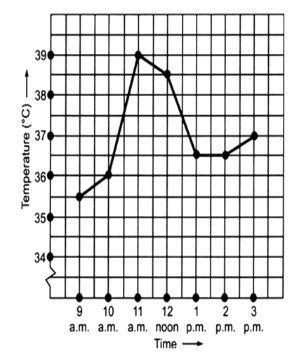(a) The patient's temperature at 1 p.m is  celsius.

When was the patients temperature(b) The patients temperature is  at 12 noon.(c)  The patients temperature was the same two times during the period given these two times are 1 p.m. and 2 p.m.

What was the temperature at 1:30 p.m? How did you arrive at your answer?(d) The temperature  at 1:30 p.m is  celsius. The temperature at 1 p.m. to 2 p.m. is same  i.e. celsius so it is also same as at 1:30 p.m is  celsius.

During which periods did the patients temperature showed an upward trend ?(e) The patients temperature showed an upward trend-  9 am to 10 am., 10 am. to 11 am. and 2pm to 3pm

What are the sales in (1) 2002  (2) 2006 ?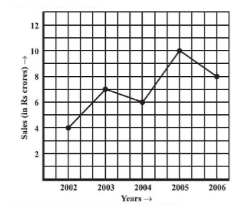(a) The sales in

(1) 2002  = 4 crore

(2) 2006 = 8 crore

What are the sales in (1) 2003  (2) 2005 ?(b) The sales in

(1) 2003  = 7 crore

(2) 2005  = 10 crore

Compute the difference between sales in 2002 and 2006(c) The difference between sales in 2002 and 2006  .

In which year was there the greatest difference between the sales as compared to its previous year  ?(d) The greatest difference between the sales as compared to its previous year is  between 2004 and 2005.

How high was Plant A after (i) 2 weeks (ii) 3 weeks?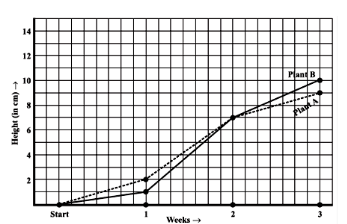(a) The Plant A after

(i) 2 weeks  = 7 cm height.

(ii) 3 weeks = 9 cm height.(b) The Plant B after

(i) 2 weeks = 7 cm height.

(ii) 3 weeks = 10cm height.

How much did Plant A grow during the 3rd week?(c)The Plant A grow during the 3rd week =

How much did Plant B grow from the end of the 2nd week to the end of the 3rd week?(d)The Plant B grow from the end of the 2nd week to the end of the 3rd week  height.

During which week did Plant A grow most?(e)The week in which Plant A grow most during 2nd week = cm height.

During which week did Plant B grow least?(f) The week in which Plant B grow least is during first week =

Were the two plants of the same height during any week shown here? Specify.(g) At the end of second week, heights of both plants are same.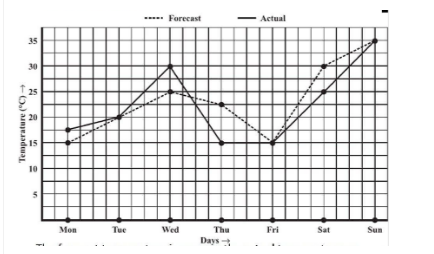(a)  The forecast temperature is the same as the actual temperature on Tuesday, Friday and Sunday.

What was the maximum forecast temperature during the week?(b) The maximum forecast temperature during the week was   on sunday.

What was the minimum actual temperature during the week ?(c) The minimum actual temperature during the week  is  .

On which day did the actual temperature differ the most from the forecast temperature ?(d) The actual temperature differs the most from the forecast temperature on Thursday.(a) The linear graph of data given above is as shown :Question:5(b) Use the tables below to draw linear graphs.

Population (in thousands) of men and women in a village in different years.(b) The graph of data given above is as shown :

Taking years as x-axis and population as y- axis.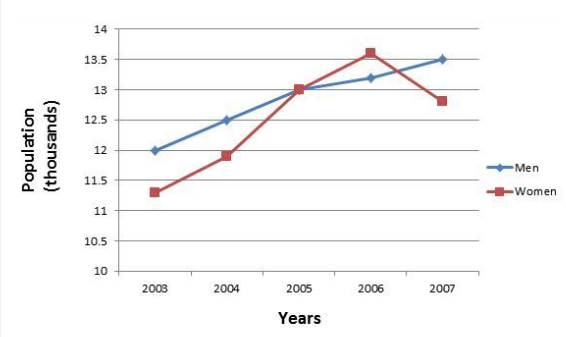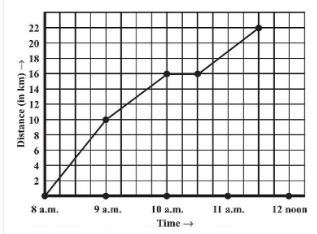(a) The scale taken for the time axis (x-axis) is 4 units = 1 hour.

How much time did the person take for the travel ?(b) The person travels from 8 a.m. to 11:30 a.m.

the person travels  hours.

How far is the place of the merchant from the town  ?(c) The place of the merchant from the town is 22 km away.

Did the person stop on his way? Explain.(d) From 10 a.m. to 10:30 a.m. ,there is a horizontal line which states that person stops from 10 a.m. to 10:30 a.m.

During which period did he ride fastest ?(e) From graph we can observe person travels maximum distance from 8 a.m. to 9 a.m.

Hence,person travels fastest from 8 a.m. to 9 a.m.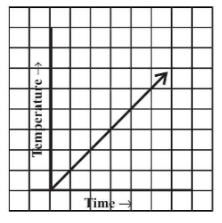(i)  Yes, there can be a time-temperature graph as shown with the increase in time, temperature also increases.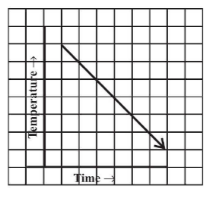(ii) Yes, it is possible that with increase in time temperature decreases.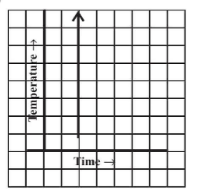(iii) No,this type of graph is not possible because different temperature at same time are not posiible.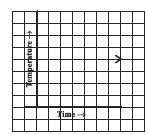(iv) Yes, it is possible i.e. same temperature at different time  is possible.

## NCERT solutions for class 8 maths chapter 15 introduction to graphs-Exercise: 15.2

A(4, 0), B(4, 2), C(4, 6), D(4, 2.5)

(a) A(4, 0), B(4, 2), C(4, 6), D(4, 2.5)P(1, 1), Q(2, 2), R(3, 3), S(4, 4)

(b) P(1, 1), Q(2, 2), R(3, 3), S(4, 4)

The graph is as shown :K(2, 3), L(5, 3), M(5, 5), N(2, 5)

(iii) K(2, 3), L(5, 3), M(5, 5), N(2, 5)

The graph is as shown :As shown below is graph :The coordinates of the points at which this line meets the x-axis and y-axis are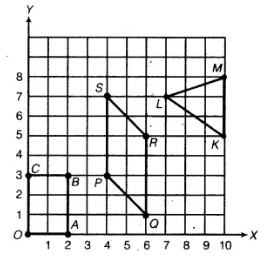The coordinates of vertices are:

, ,,

,,,

,,

(i) True .A point whose x coordinate is zero and y-coordinate is non-zero will
lie on the y-axis. =  here, y is variable.

Question:4(ii) State whether True or False. Correct that are false.

A point whose y coordinate is zero and x-coordinate is 5 will lie on y-axis.

(ii) False.Point  does not lie on y-axis.A point whose y coordinate is zero and x-coordinate is 5 will not lie on
y-axis.

Question:4(iii) State whether True or False. Correct that are false.

The coordinates of the origin are (0, 0).

(iii)True. The coordinates of the origin are (0, 0).

NCERT solutions for class 8 maths chapter 15 introduction to graphs topic 15.3 some applications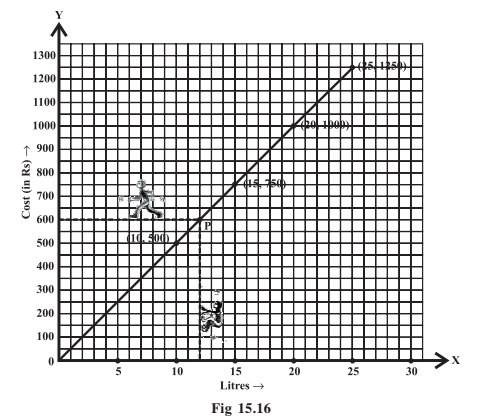From the graph given below we can say that for  Rs 800 we can buy 16 litres.Taking a case of direct variation the graph is as shown below.

Taking sum deposite as 100,200,300,500 ,1000.

and  10% Simple Interest (S.I.).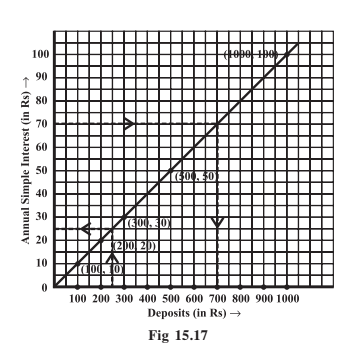NCERT solutions for class 8 maths chapter 15 Introduction to Graphs-Exercise: 15.3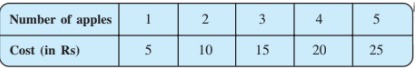(a) Cost of apples(i)How much distance did the car cover during the period 7.30 a.m. to 8 a.m?(b)(i)From the above graph,the distance the car cover during the period 7.30 a.m. to 8 a.m is

Distance travelled by a car.(ii) What was the time when the car had covered a distance of 100 km since it’s star

(b)(ii)From above graph we conclude that at 7:30 a.m.,  the car had covered a distance of 100 km since it’s start.

Question:1(c) Interest on deposits for a year .(i) Does the graph pass through the origin?

(c)Taking x axis as 1000 units = 1 box and y axis as 100 units = 1 box.

(i)Yes, the graph pass through the origion.

Question:1(c) Interest on deposits for a year(ii) Use the graph to find the interest on Rs 2500 for a year.

(c)(ii) Using the graph, the interest on Rs 2500 for a year is Rs 200.

Question:1(c) Interest on deposits for a year .(iii) From the graph,to get an interest of Rs 280 per year  Rs 3500 money should be deposited.

Question:2(i) Draw a graph for the following Is it a linear graph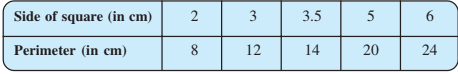From the above graph ,we conclude that graph is linear.

Question:2(ii) Draw a graph for the following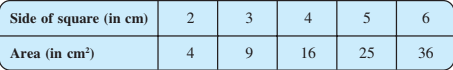Is it a linear  graph?From above graph,we conclude that graph is not linear.

## NCERT solutions for class 8 maths: Chapter-wise

 Chapter -1 NCERT solutions for class 8 maths chapter 1 Rational Numbers Chapter -2 Solutions of NCERT for class 8 maths chapter 2 Linear Equations in One Variable Chapter-3 CBSE NCERT solutions for class 8 maths chapter 3 Understanding Quadrilaterals Chapter-4 NCERT solutions for class 8 maths chapter 4 Practical Geometry Chapter-5 Solutions of NCERT for class 8 maths chapter 5 Data Handling Chapter-6 CBSE NCERT solutions for class 8 maths chapter 6 Squares and Square Roots Chapter-7 NCERT solutions for class 8 maths chapter 7 Cubes and Cube Roots Chapter-8 Solutions of NCERT for class 8 maths chapter 8 Comparing Quantities Chapter-9 NCERT solutions for class 8 maths chapter 9 Algebraic Expressions and Identities Chapter-10 CBSE NCERT solutions for class 8 maths chapter 10 Visualizing Solid Shapes Chapter-11 NCERT solutions for class 8 maths chapter 11 Mensuration Chapter-12 Solutions of NCERT for class 8 maths chapter 12 Exponents and Powers Chapter-13 CBSE NCERT solutions for class 8 maths chapter 13 Direct and Inverse Proportions Chapter-14 NCERT solutions for class 8 maths chapter 14 Factorization Chapter-15 NCERT solutions for class 8 maths chapter 15 Introduction to Graphs Chapter-16 CBSE NCERT solutions for class 8 maths chapter 16 Playing with Numbers

## Benefits of NCERT solutions for class 8 maths chapter 15 introduction to graphs-

• You will get to know the different applications of the graph based on the need and given data.
• You can predict future events with the help of graphs using data of past events.
• You will also get solutions to the practice questions given below every topic which will give you conceptual clarity.
• You should draw the graphs given in the NCERT textbook. You can take help from the NCERT solutions for class 8 maths chapter 15 introduction to graphs.

Happy learning!!!